Howdy, Stranger!

It looks like you're new here. If you want to get involved, click one of these buttons!

Options

Lecture 51 - Chapter 3: Right Kan Extensions

edited July 2018

Last time we built new databases from old using a left Kan extension. Let's compare how a right Kan extension works in the exact same situation! Having a good feel for the difference between left and right adjoints, and in particular left and right Kan extensions, is one of the marks of a true category theorist. It takes a while, but the key is thinking about examples.

We saw that the left Kan extension had the ability to automatically extend a database from a small schema like this: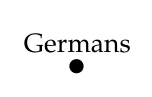to a larger one like this: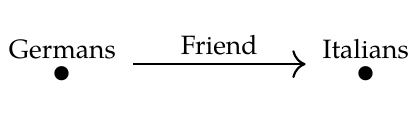This goes 'against the grain'. What's easy to do is restrict a database from a larger schema to a smaller one by discarding information - in this case, all information involving Italians. Going from a smaller schema to a larger one is hard because there's not really enough information. The left Kan extension does this by systematically creating new entries to serve as 'fillers' for missing information. In our example, it makes up a bunch of anonymous Italians to serve as friends for the Germans.

And since the left Kan extension is a left adjoint, it does this in a 'liberal' or 'generous' way. Mathematically, we say it does this in a free way. It takes a while to understand what this mean, and it's a bit hard to explain in words: the definition of left Kan extension is the way to make the idea truly precise. But roughly speaking, the left Kan extension freely makes up all the entries that are needed, obeying only the equations that are needed to get a valid database. For example, if you hand Mr. Left Kan Extension this database: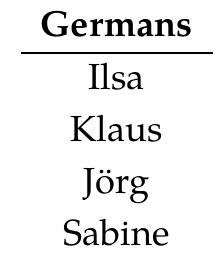it will create this database of Germans, Italians, and which Germans have which Italians as friends: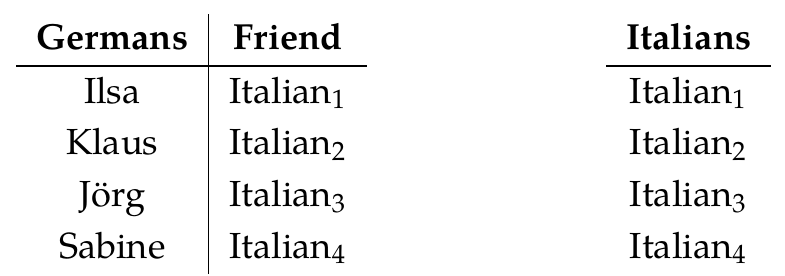So, it makes up only the Italians that are needed for a valid database - one to be a friend of each German - and it doesn't make any of these Italians the same as any other, because that's not required.

The right Kan extension is another systematic way of solving the same problem. Since it's a right adjoint, it does this in a 'conservative' or 'cautious' way. In the same example, it creates this database: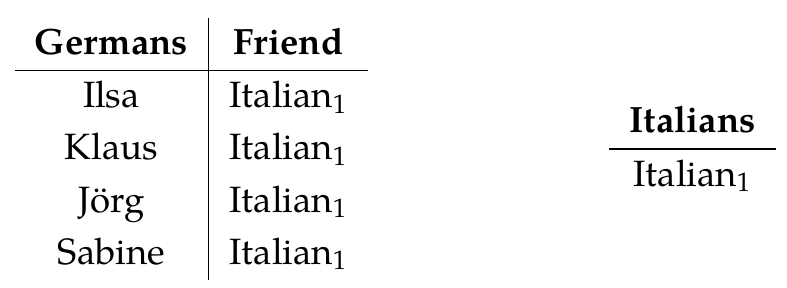This time it makes up just one Italian! In general, the right Kan extension makes up all the entries that are possible, but imposes all the equations that are possible in a valid database.

Let's look at the general theory a bit more. As before, we start with two categories $$\mathcal{C}$$ and $$\mathcal{D}$$, which serve as database schemas. Then we choose a functor $$G: \mathcal{D} \to \mathcal{C}$$. This gives a process for turning any database built using the first schema, say

$$F: \mathcal{C} \to \mathbf{Set}$$ into a database built using the second schema, namely

$$F \circ G : \mathcal{D} \to \mathbf{Set} .$$ This process is actually a functor:

$$\text{composition with } G : \mathbf{Set}^\mathcal{C} \to \mathbf{Set}^\mathcal{D} .$$ The left and right Kan extensions are two ways to reverse this process: they are the left and right adjoints of the above functor!

Today we're looking at the right Kan extension. This is defined as follows: there's a natural one-to-one correspondence between

$$\textrm{natural transformations } \alpha: F \circ G \Rightarrow H$$ and

$$\textrm{natural transformations } \beta: F \Rightarrow \textrm{Ran}_G(H) .$$ Let's see how it works in our example. We're taking $$\mathcal{D}$$ to be the free category on this graphand $$\mathcal{C}$$ to be the free category on this bigger graph:We're taking $$G : \mathcal{D} \to \mathcal{C}$$ to be the obvious inclusion of $$\mathcal{D}$$ in the bigger category $$\mathcal{C}$$.

If we take $$F : \mathcal{C} \to \mathbf{Set}$$ to be this database: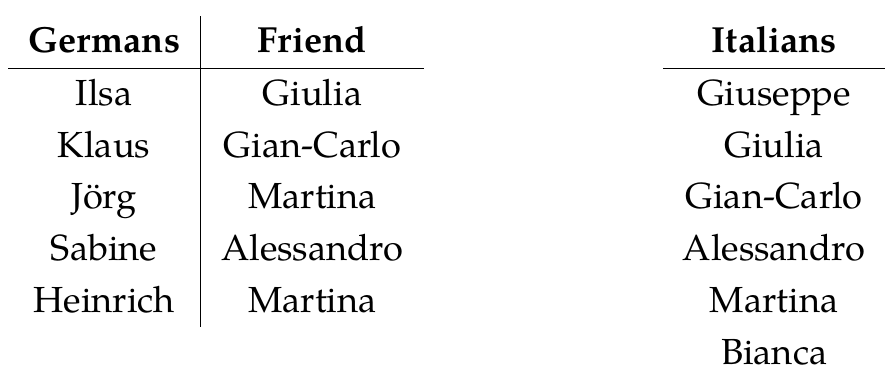then $$F \circ G$$ is this database: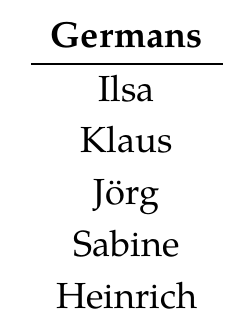So, it's just the restriction of $$F$$ to the smaller category $$\mathcal{D}$$.

But suppose we want to reverse this process! We start with a functor defined on the smaller category, say $$H : \mathcal{D} \to \mathbf{Set}$$. This is a database built on our smaller schema:Then we extend it to the larger category using a right Kan extension, getting a functor $$\textrm{Ran}_G(H): \mathcal{C} \to \mathbf{Set}$$. This gives a database built on our larger schema, and I claim it looks like this:Can you check this? It's just a matter of patiently unraveling all the definitions! I won't make you prove that $$\textrm{Ran}_G(H)$$ really meet all the conditions of a right Kan extension, just that it seems to be on the right track.

Puzzle 160. We know from the definition of right Kan extension that there should be a natural one-to-one correspondence between

$$\textrm{natural transformations } \alpha: F \circ G \Rightarrow H$$ and

$$\textrm{natural transformations } \beta: F \Rightarrow \textrm{Ran}_G(H) .$$ Can you guess how it works for our particular choice of $$F,G$$ and $$H$$? By counting the number of natural transformations of the two kinds you can show that there exists a one-to-one correspondence between them, but there should be an 'obvious best' one-to-one correspondence.

To read other lectures go here.

• Options
1.
edited June 2018

Below is a diagram of the right Kan Extension. Then I drew both left and right extensions in one diagram to emphasize the difference.Puzzle 160There are $$4^5$$ natural transformations for both $$\alpha$$ and $$\beta$$.

Edit: I mislabeled the category of $$H$$ and $$F \circ G$$. Now its fixed.

Comment Source:Below is a diagram of the right Kan Extension. Then I drew both left and right extensions in one diagram to emphasize the difference. ![Right Kan Extension](http://aether.co.kr/images/right_kan_extension.svg) ![Left and Right Kan Extension](http://aether.co.kr/images/left_right_kan_extension.svg) **Puzzle 160** ![Right Kan Extension natural transformation](http://aether.co.kr/images/kan_extension_example_2.svg) There are \$$4^5\$$ natural transformations for both \$$\alpha\$$ and \$$\beta\$$. Edit: I mislabeled the category of \$$H\$$ and \$$F \circ G\$$. Now its fixed.
• Options
2.
edited June 2018

Michael, your lower diagram has $$H$$ and $$F\circ G$$ mislabeled as taking $$\mathcal{C} \to \mathbf{Set}$$ instead of $$\mathcal{D} \to \mathbf{Set}$$.

Comment Source:Michael, your lower diagram has \$$H\$$ and \$$F\circ G\$$ mislabeled as taking \$$\mathcal{C} \to \mathbf{Set}\$$ instead of \$$\mathcal{D} \to \mathbf{Set}\$$.
• Options
3.

Great pictures, Michael!

Can someone explain in words the bijection between

$$\textrm{natural transformations } \alpha: F \circ G \Rightarrow H$$ and

$$\textrm{natural transformations } \beta: F \Rightarrow \textrm{Ran}_G(H) ?$$ Michael correctly claims there are $$4^5$$ of each, but there is some wisdom to be gained by understanding the bijection... since this will help us with more complicated right Kan extensions.

Comment Source:Great pictures, Michael! Can someone explain in words the bijection between $\textrm{natural transformations } \alpha: F \circ G \Rightarrow H$ and $\textrm{natural transformations } \beta: F \Rightarrow \textrm{Ran}_G(H) ?$ Michael correctly claims there are \$$4^5\$$ of each, but there is some wisdom to be gained by understanding the bijection... since this will help us with more complicated right Kan extensions.
• Options
4.

It keeps the effect on germans, and maps all italians to a single point. While mapping the function to the unique function to the terminal object.

Comment Source:It keeps the effect on germans, and maps all italians to a single point. While mapping the function to the unique function to the terminal object.
• Options
5.
edited June 2018

I am not sure if this is completely correct but using the hints John gave us and continuing Christopher's explanation, the bijection is giving priority to preserving the relations the best it can. For example, Heinrich and Jörg both map to Martina. For the left extension, this relation doesn't get preserved. But in the right extension, it does get preserved although it is has been completely dumbed down due to the loss of information,.

Comment Source:I am not sure if this is completely correct but using the hints John gave us and continuing Christopher's explanation, the bijection is giving priority to preserving the relations the best it can. For example, Heinrich and J&ouml;rg both map to Martina. For the left extension, this relation doesn't get preserved. But in the right extension, it does get preserved although it is has been completely dumbed down due to the loss of information,.
• Options
6.
edited June 2018

Working on the two explanations given above, the natural transformation $$\beta_\text{Italians}$$ maps every Italian in $$F$$ to the Italian in $$\mathrm{Ran}_G (H)$$, of which there can be only one unique possible function.

We can therefore reason that $$\beta_\text{Germans}$$ and $$\alpha_\text{Germans}$$ are effectively the same map, where the sizes of each are equated by \begin{align} |\alpha_\text{Germans}| \\ = |F\circ G(\text{Germans})|^{|H(\text{Germans})|} \\ = 4^5 \\ = |F(\text{Germans})|^{|Ran_G(H)(\text{Germans})|} \\ = |\beta_\text{Germans}|. \end{align}

Comment Source:Working on the two explanations given above, the natural transformation \$$\beta\_\text{Italians}\$$ maps *every* Italian in \$$F\$$ to *the* Italian in \$$\mathrm{Ran}\_G (H)\$$, of which there can be only one unique possible function. We can therefore reason that \$$\beta\_\text{Germans}\$$ and \$$\alpha\_\text{Germans}\$$ are effectively the same map, where the sizes of each are equated by \\begin{align} |\alpha\_\text{Germans}| \\\\ = |F\circ G(\text{Germans})|^{|H(\text{Germans})|} \\\\ = 4^5 \\\\ = |F(\text{Germans})|^{|Ran\_G(H)(\text{Germans})|} \\\\ = |\beta\_\text{Germans}|. \end{align} \
• Options
7.
edited July 2018

By the way, I had things backwards here:

We're taking $$\mathcal{C}$$ to be the free category on this graphand $$\mathcal{D}$$ to be the free category on this bigger graph:I've fixed it. The lecture now reads:

We're taking $$\mathcal{D}$$ to be the free category on this graphand $$\mathcal{C}$$ to be the free category on this bigger graph:Comment Source:By the way, I had things backwards here: > We're taking \$$\mathcal{C}\$$ to be the free category on this graph > <center><img width = "270" src = "http://math.ucr.edu/home/baez/mathematical/7_sketches/graph_database_5.png"></center> > and \$$\mathcal{D}\$$ to be the free category on this bigger graph: > <center><img width = "100" src = "http://math.ucr.edu/home/baez/mathematical/7_sketches/graph_database_6.png"> </center> I've fixed it. The lecture now reads: > We're taking \$$\mathcal{D}\$$ to be the free category on this graph > <center><img width = "100" src = "http://math.ucr.edu/home/baez/mathematical/7_sketches/graph_database_6.png"></center> > and \$$\mathcal{C}\$$ to be the free category on this bigger graph: > <center><img width = "270" src = "http://math.ucr.edu/home/baez/mathematical/7_sketches/graph_database_5.png"></center>